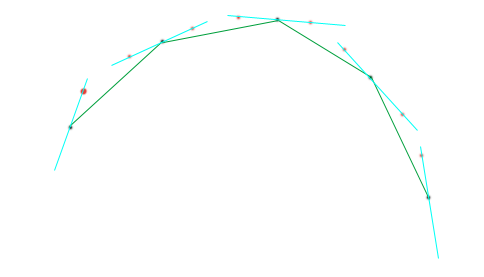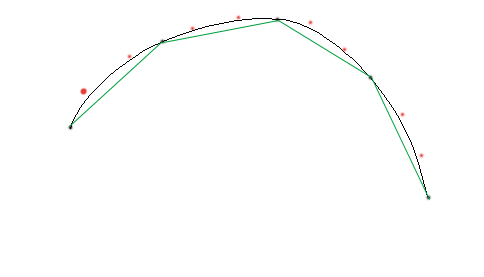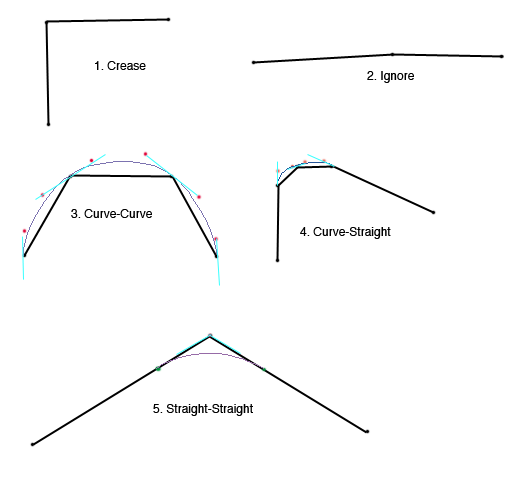## Saturday, September 03, 2011

### Bezier Curve Optimization

I've been meaning to write up a few notes about how we turn OSM vector data into bezier curves for X-Plane.  The code is all open source - look in the scenery tools web code browser at NetPlacement.cpp and BezierApprox.cpp for the code.

First, a few basics:
• OSM vector data comes as a polyline data - that is, each road is a series of points connected by straight line segments.  There are a lot of points - sometimes every few meters along a road.
• X-Plane 10 uses piece-wise bezier curves, meaning a road is a string of end-to-end bezier curves.  Each curve can be a line segment, quadratic, or cubic bezier curver, but not anything of higher degree.
• The representation in X-Plane for the piece-wise bezier curves is a list of "tagged" points, where the tag defines whether a point is a piece-wise curve end-point or control point.  Semantically, the two end points must not be control points (must not be tagged) and we can never have more than two consecutive control points (because that would define a higher-order bezier).
• There is no requirement that the curve be smooth - we can create a sharp corner at any non-control point, even between two bezier curves.
Both OSM and X-Plane's road system have network topology, but for the purpose of converting a polyline to a piece-wise bezier curve we care only about a single road between junctions - that is, a "curve" in topology-terms.

We divide the problem into two parts: converting the polyline to a piece-wise bezier curve and optimizing that bezier curve to reduce point count.

To build the initial beziers we take an idea from ATI's PN-Triangles paper. The basic idea from the paper is this: if we have a poly-line (or triangle mesh) approximation of a curved surface, we can estimate the tangents at the vertices by averaging the direction of all incident linear components.  With the tangents at the vertices, we can then construct a bezier surface through that tangent (because a bezier curve's tangent at its end point runs toward the next control point) and use that to "round out" the mesh.

The idea is actually a lot easier to understand for polylines, where the dimensionality is lower.  For each vertex in our road, we'll find the "average" direction of the road (the tangent line) at that vertex based on the two segments coming into that vertex.  The bezier control points adjacent to the vertex must run along that tangent line; we can then adjust the distance of the control points from the end point to control the amount of "bulge".We start with a poly-line.We calculate the tangents at each vertex.We place bezier control points along the tangents at fixed fractional lengths.The result is a smooth piece-wise approximation through every point.
PN triangles tends to make meshes "bulge", because a curve around a convex hull always extends outward.  You can see the same look in our interpolation.  This is good for on-ramps but actually looks quite bad for a straight-away road - the road "bulges out" when the curve ends.

To address this, we categorize a road as "straight" if the road is long enough that if we built a curve out of it, the radius of that curve would be larger than a constant value.  (We pick different constants for different types of roads.)  In other words, if two highway segments are each 1 km long and they meet at a 3 degree angle, we do not assume it is part of an arc with a 19 km radius - we assume that most of the 1 km road are straight, with a small curve (of much smaller radius) at the end.  For any given point, we can decide whether either one or both of the two adjoining line segments is "straight" or should be entirely curved.  We then form five cases:
1. If two segments come together at a very sharp angle, we simply keep the sharp angle.  We assume that if the data had this sharp angle in the original vector data (which is quite highly noded) then there really is some kind of sharp corner.
2. If the two segments come together at a very shallow angle, we simply keep the corner, because who cares.  This case matters when we have a very tiny angle (e.g. 0.25 degrees) but very long line segments, such that removing the tiny angle would cause significant change in the vector position due to the long "arm" and not the steep angle.  We trust that for our app the tiny curve isn't going to be visible.
3. If the two segments are both curved, we use the PN-triangle-style tangents as usual.
4. If one of the segments is curved and one is straight, the tangent at the point comes from the straight curve.  This takes the "bulge" out of the beginning and ending of our curves by ensuring that the curve ends by heading "into" the straight-away.
5. If both segments are straight, we need to round the corner on the inside.  We do this by pulling back the corner along both curves and using the original point as a quadratic bezier control point.
One note about cases 2 and 5: if you pull back a vertex proportional to the angle of the vertex, then very small angles can result in very small pull-backs, resulting in a very short curve.  In the case of X-Plane, we want to ensure that there is a minimum distance between points (so that rounding errors in the DSF encode don't give us colocated points); this means that the minimum angle for case 2 has to be large enough to prevent a "tiny curve" in case 5.The five curve cases, illustrated.
With these five curve cases we get pretty good looking curved roads.  But our point gets out of control - at a minimum we've kept every original point, and on top of that we've added one or two bezier contrl points per segment.

What we need to do is generalize our curves.  Again, the PN-triangles observation can help us.  If we want to replace two piece-wise bezier curves with a single one, we know this: the tangent at the end of the curves can't change.  This means that the two control points of the approximate curve must be colinear with the control points of the original curve ends and the original curve ends itself.

So what?  Well, if we can only move the control points "in and out" then there are really only two scalar variables for all possible approximations: how much to scale the control handles at the start and end.  And that's something we can check with brute force!

Below is the basic step to approximating a piece-wise bezier curve with two pieces as a single cubic bezier.We start with a piece-wise bezier with nodes and control points.For each range of curves that we will simplify, we "push" the outermost control points along the tangent vector by an arbitrary scaling factor.The resulting curve will be close, but not quite the same as the original.The original also looks "reasonable" on its own - that is, the approximations tend to have good curvature characteristics.
To find the approximate curve, we simply "search" a whole range of scalar values by trying them and measuring curve error.  In the scenery tools code, we do a two-step search, refining the scalars around the values of least error.  The initial values are picked experimentally; it's almost certainly possible to do a better job of guessing scalar values but I haven't had time to research it more.

To measure the error we approximate the bezier with polylines (e.g. we turn each individual bezier into a poly-line of N segments) and then compare the polylines.  The polyline comparison is the variance of the distances of each point in one polyline to the other. (in other words, we treat one polyline as a point set and take the variance of the distance-to-polyline of each point).  This is similar to the Hausdorff distance with two key differences:
• Because we are taking variance and not a minimum error, we can't use our previous minimum distance from a point to a line segment to limit our spatial searches.  (See below.)  Instead, we pick some large distance beyond which the curves are too different and we use that to limit.  For low maximum acceptable errors this gives us good performance.
• Since the variance depends on all points and not just the worst one, we can rank multiple approximations - that is, generally better approximations score quite a bit higher.
To speed up the polyline compare (which is naively O(N^2) and dominates CPU time if implemented via a nested for-loop) we can create a spatial index for the "master" line (since we will compare many candidates to it) and search only a few of the master segments when looking for the closest one.  If we know that past a certain error we're simply broken, then we can limit our queries. For my implementation, I pick an axis (e.g. X or Y) based on the original curve shape and then subdivide the polyline into monotone sub-polylines that can be put into a coordinate-sorted map.  Then we can use lower_bound to find our segments in log(N) time.  An rtree would probably work as well.

Phew.  So we can take a piece-wise bezier and come up with the best approximation through brute force and error checking.  How do we simplify an entire road?  The answer is not Douglas-Peuker.  Instead we use a bottom-up combine:
• For every non-end node in the piece-wise curve, we build the approximation of the two adjoining bezier curves and measure its error.
• We queue every "possible merge" by error.
• Until the queue is empty or the lowest error is too large we..
• Replace the two curves in the merge by one.
• Recalculate the two neighboring merges (since one of their source curves is now quite a bit different).  Note that we must keep the original beziers around to get accurate error metrics, so a merge of two curves that originally covered eight curves is an approximation of all eight originals, not the two previous merges.
Why is this better than DP?  Well, the cost of approximating two curves is going to be O(NlogN) where N is the number of pieces in the piece-wise curve - this comes straight from the cost of error measurement.  Therefore the first run through DP, just to add one point back, is going to be O(N^2logN) because it must do a full curve comparison between the original and every pair it tries.  When the curve approximation is going to contain a reasonably large number of pieces (e.g. we might merge each bezier with its neighbor and be done) the bottom-up approach gets there fast while DP does a ton of work just to realize that it didn't need to do that work.  (DP doesn't have this issue with polylines because the comparison cost is constant per trial point.)

## Thursday, September 01, 2011

### Sequences Vs. Iterators

Lately I've been playing with an STL "sequence" concept.  That's a terrible name and I'm sure there are other things that are officially "sequences" (probably in Boost or something) and that what I am calling actually have some other name (again, probably in Boost).  Anyway, if you know what this stuff should be called, please leave a comment.

EDIT: Arthur pointed me to this paper; indeed what I am calling sequences are apparently known as "ranges".  Having just recoded victor airway support and nav DB access using ranges, I can say that the ability to rapidly concatenate and nest filters using adapter templates is a huge productivity win.  (See page 44 to have your brain blown off of its hinges.  I thought you needed Python for that kind of thing!)

Basically: a sequence is a data type that can move forward, return a value, and knows for itself that it is finished - it is the abstraction of a C null-terminated string.  Sequences differ from forward iterators in that you don't use a second iterator to find the end - instead you walk the sequence until it ends.

Why would you ever want such a creature?  The use case that really works nicely is adaptation.  When you want to adapt an iterator (e.g. wrap it with another iterator that skips some elements, etc.) you need to give your current iterator both an underlying "now" iterator and the end; similarly, the end iterator you use for comparison is effectively a place-holder, since the filtered iterator already knows where the end is.

With sequences life is a lot easier: the adapting sequence is done when its underlying sequence is done.  Adapting sequences can easily add elements (e.g. adapt a sequence of points to a sequence of mid points) or remove them (e.g. return only sharp corners).

My C++ sequence concept uses three operators:
• Function operator with no arguments to tell if the sequence is still valid - false means it is finished.
• Pre-increment operator advances to the next item.
• Dereference operator returns the current value.
You could add more - post increment, copy constructors, comparisons, etc. but I'm not sure that they're necessary.  The coding idiom looks like this:

while(my_seq()) {     do_stuff_to(*my_seq);     ++my_seq; }